Test: Amplifiers

# Test: Amplifiers - Electrical Engineering (EE)

Test Description

## 10 Questions MCQ Test GATE Electrical Engineering (EE) 2023 Mock Test Series - Test: Amplifiers

Test: Amplifiers for Electrical Engineering (EE) 2023 is part of GATE Electrical Engineering (EE) 2023 Mock Test Series preparation. The Test: Amplifiers questions and answers have been prepared according to the Electrical Engineering (EE) exam syllabus.The Test: Amplifiers MCQs are made for Electrical Engineering (EE) 2023 Exam. Find important definitions, questions, notes, meanings, examples, exercises, MCQs and online tests for Test: Amplifiers below.
Solutions of Test: Amplifiers questions in English are available as part of our GATE Electrical Engineering (EE) 2023 Mock Test Series for Electrical Engineering (EE) & Test: Amplifiers solutions in Hindi for GATE Electrical Engineering (EE) 2023 Mock Test Series course. Download more important topics, notes, lectures and mock test series for Electrical Engineering (EE) Exam by signing up for free. Attempt Test: Amplifiers | 10 questions in 30 minutes | Mock test for Electrical Engineering (EE) preparation | Free important questions MCQ to study GATE Electrical Engineering (EE) 2023 Mock Test Series for Electrical Engineering (EE) Exam | Download free PDF with solutions
 1 Crore+ students have signed up on EduRev. Have you?
Test: Amplifiers - Question 1

### What is the output waveform of the circuit?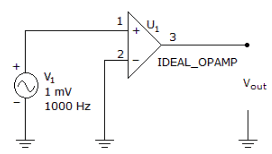Test: Amplifiers - Question 2

### Consider the circuit of fig. The transistor parameters are  β = 120 and VA = ∞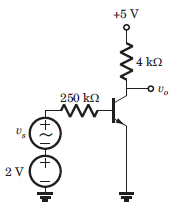Que: The hybrid-π parameter values of gm ,rπ  and ro are

Detailed Solution for Test: Amplifiers - Question 2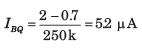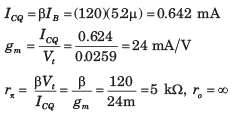Test: Amplifiers - Question 3

### Consider the circuit of fig. The transistor parameters are  β = 120 and VA = ∞Que: The small signal votlage gain Av = vo/vs

Detailed Solution for Test: Amplifiers - Question 3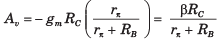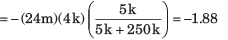Test: Amplifiers - Question 4

The nominal quiescent collector current of a transistor is 1.2 mA. If the range of β for this transistor is 80 ≤ β ≤ 120 and if the quiescent collector current changes by +-10 percent, the range in value for rπ is

Detailed Solution for Test: Amplifiers - Question 4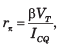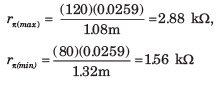Test: Amplifiers - Question 5

Consider the circuit in fig. The transistor parameter are β = 100 and VA = ∞.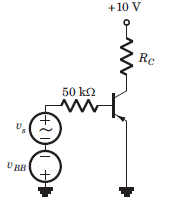If Q-point is in the center of the load line and ICQ = 0.5 mA, the values of VBB and RC are

Detailed Solution for Test: Amplifiers - Question 5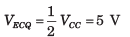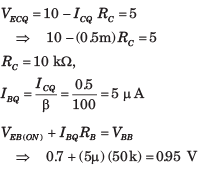Test: Amplifiers - Question 6

Consider the common Base amplifier shown in fig. The parameters are β = 100, gm = 2 mS and ro = 250 kΩ. Find the Thevenin equivalent faced by load resistance RL .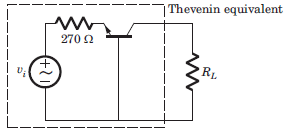Que: The Thevenin voltage vTH is

Detailed Solution for Test: Amplifiers - Question 6

The equivalent circuit is shown below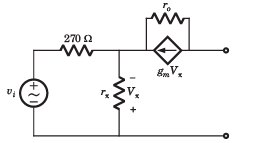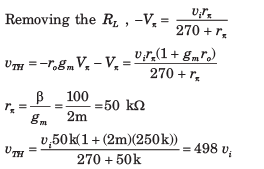Test: Amplifiers - Question 7

Consider the common Base amplifier shown in fig. The parameters are gm = 2 mS and ro = 250 kΩ. Find the Thevenin equivalent faced by load resistance RL .Que: The Thevenin equivalent resistance RTH is

Detailed Solution for Test: Amplifiers - Question 7

The equivalent small-signal circuit is shown in fig.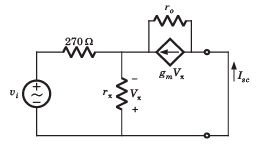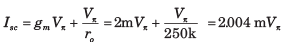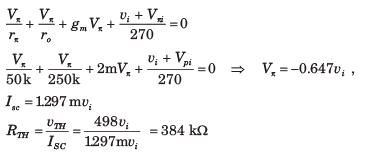Test: Amplifiers - Question 8

The common-base amplifier is drawn as a two-port in fig. The parameters are β =100, gm = 3 mS, and ro =  800 kΩ.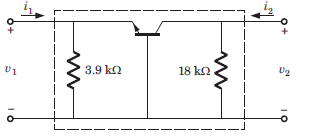Que: The h-parameter h21 is

Detailed Solution for Test: Amplifiers - Question 8

The equivalent small-signal circuit is shown in fig.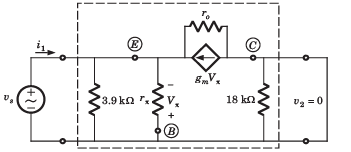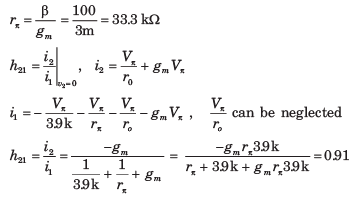Test: Amplifiers - Question 9

The common-base amplifier is drawn as a two-port in fig. The parameters are β =100, gm = 3 mS, and ro =  800 kΩ.Que: The h-parameter h12 is

Detailed Solution for Test: Amplifiers - Question 9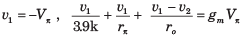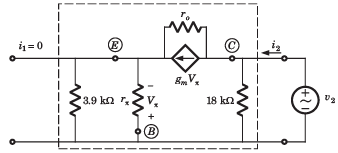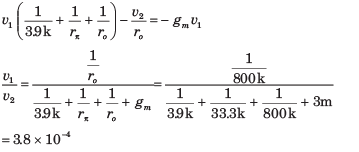Test: Amplifiers - Question 10

Consider the source-follower circuit in fig. The values of parameter are gm = 2 mS and ro = 100 kΩ.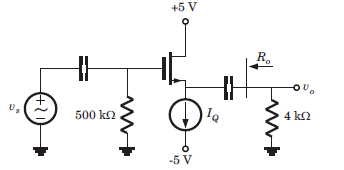Que: The voltage gain Av is

Detailed Solution for Test: Amplifiers - Question 10

The small-signal equivalent circuit is shown in fig.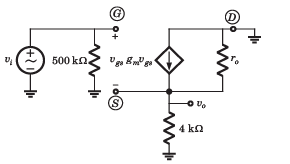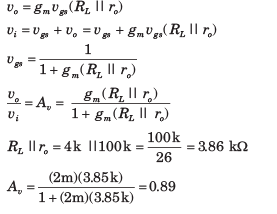## GATE Electrical Engineering (EE) 2023 Mock Test Series

22 docs|284 tests(Scan QR code)